## Want to keep learning?

This content is taken from the The Open University & Persontyle's online course, Advanced Machine Learning. Join the course to learn more.
3.10

# Gaussian Processes

We now introduce another very popular kernel method: Gaussian processes. As well as being used for regression problems it is commonly used in Bayesian non-parametric statistics, when we wish to sample from a probability distribution over functions.

#### Aside: Kriging

Gaussian processes have received a lot of attention from the machine learning community over the last decade. However they were originally developed in the 1950s in a master thesis by Danie Krig, who worked on modeling gold deposits in the Witwatersrand reef complex in South Africa. The mathematics was formalized by the French mathematician Georges Matheron in 1960. The method developed was known as kriging, and this term remains common in geo-statistics literature.

Gaussian processes are simple to understand, but require one important change of perspective. The change of perspective is this: We consider the values of the target variable to be random variables whose multivariate probability distribution is a multivariate Gaussian. The mean of this multivariate Gaussian is typically 0 (if this is unreasonable, we can center the target variables so that their sample mean is 0), and the covariance matrix, $$\Sigma$$ is a function of the input features such that $$\Sigma_{i,j}=k(X_i,X_j)$$, where $$k$$ is some kernel function.

Consider the following training data:

$$X$$ $$Y$$
4.5 2.1
1.6 4.3
3.5 1.7

We assume a mean of 0, and use the kernel $$k=e^{- \| X_1,X_2 \|}$$ to generate the covariance matrix. Notice that the $$k(X_i,X_j)==1$$ when $$X_i=X_j$$ and $$k(X_i,X_j) \to 0$$ as $$\|X_i-X_j\| \to \infty$$. So the value falls off as the distance between two input features increase, which is exactly what we want since we can assume that the covariance between target values depends on the nearness of their input features. The kernel we use should be one that accurately reflected the covariance of $$Y$$-values based on their distance in the input space. Calculating $$\Sigma$$ we get:

$\Sigma = \begin{bmatrix} 1 & .055 & .3679 \\ .055 & 1 & .1496 \\ .3679 & .1496 & 1 \end{bmatrix}$

Where, for example:

$\sigma_{11}=k(X_1,X_2)=e^{-\|4.5-1.6\|}=e^{-2.9}=.055$

Using $$Y_{1:3}$$ as shorthand for $$Y_1,Y_2,Y_3$$ (and similarly for $$X$$) and letting $$x_{1:3} = \langle 4.5,1.6,3.5 \rangle$$, our model is:

$P(Y_{1:3}|X_{1:3}=x_{1:3}) = \mathcal{N} (0,\Sigma)$

To understand how such a model can work, consider how we would use such a model to estimate the target value of a new input feature, $$X_* = .37$$. Since the covariance matrix is a function only of the input features, we can generate the coveriance matrix, $$\Sigma_*$$, for $$X \cup X_*$$:

$\Sigma_* = \begin{bmatrix} 1 & .055 & .3679 & .0161\\ .055 & 1 & .1496 & .2923\\ .3679 & .1496 & 1 & .0437\\ .0161 & .2923 & .0437 & 1\\ \end{bmatrix}$

And we have the joint distribution for $$Y_{1:3},Y_*$$:

$P(Y_{1:3,*} | X_{1:3,*}=x_{1:3,*}) = \mathcal{N} (0,\Sigma_*)$

If $$y_{1:3}= \langle 2.1,4.3,1.7 \rangle$$ then we want to find:

$P(Y_*|X_{1:3,*}=x_{1:3,*},Y_{1:3}=y_{1:3}) = \mathcal{N} (\mu_*,\sigma_*^2)$

There are closed form formulas for calculating conditional multivariate Gaussian distributions (if you are interested, see aside below), meaning that we can quickly calculate this conditional distribution. We will be able to find both the expected value ($$\mu_*$$) and variance ($$\sigma_*^2$$) of $$\hat{Y}_*$$.

Aside: The interested student can see the process for calculating conditional multivariate Gaussian distributions in the Calculating conditional multivariate Gaussian distributions document available in the downloads section at the end of this article.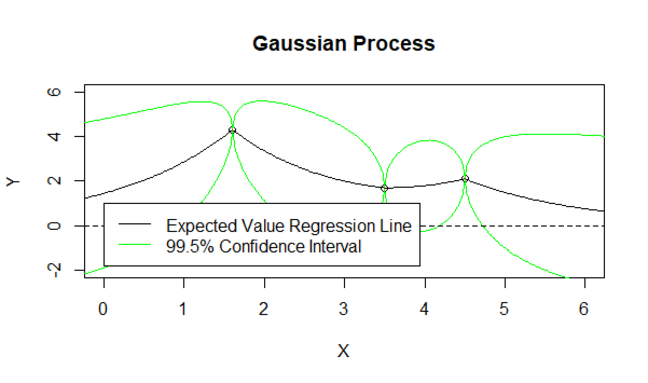We examine this model graphically. We see that it produces a regression curve, with confidence intervals around it. The confidence intervals here are chosen to be three times the standard deviation at each point, which equates to approximately 99.5% confidence (we estimate that the true values of Y at each X point lies within this interval with .995 probability). Note the confidence intervals vanish to 0 at the data points (something which we will return to below), and expand as we get further away from training examples.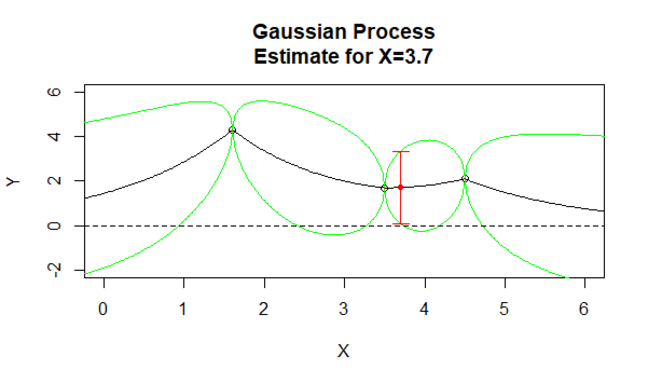For our new point, we obtain both the expected value, and the confidence intervals around it. These confidence intervals are calculated from the variance (i.e. the squared standard deviation) estimate of $$\hat{Y}_*$$, using the process explained above.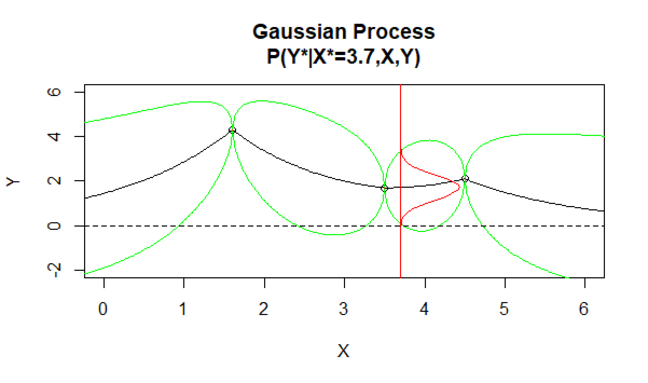Of course, what we really receive is a probability distribution for the value of $$Y$$ at $$X=3.7$$. The confidence intervals are simply a means of displaying an interval of sufficient confidence for the problem we are modeling: 99.5% of the density of the (vertically) displayed probability distribution lies between the confidence intervals.

If you are wondering how we get the regression and confidence interval curves, like any function curves, these are simply generated for each X value (in reality a sequence of X values and then joined).

#### Aside: Standard Deviations and Probability Density

For a Gaussian distribution, we know the proportion of the probability density that lies within an interval of a particular number of standard deviations centered at the mean. This allows a simple way of generating confidence intervals (though using the probability density directly is not significantly more difficult). Commonly used intervals are:

$$\mu \pm n \sigma$$ $$\approx$$ density proportion Used for
$$n=1$$ .6827 .68
$$n=2$$ .9545 .95
$$n=3$$ .9973 .995

## Variance at the training points

We noted that the variance at training points drops to 0. This means that we are sure that if we see an $$X$$ value that we had in our training data, we are certain that the corresponding $$Y$$ value will match that of the training example exactly. Except for the rare case of modeling mathematical functions, this is unreasonable.

We can avoid this by introducing a hyper-parameter, $$\lambda$$, which is added to the diagonal of the kernel matrix when calculating the covariance matrix, $$\Sigma$$. This leads to:

$\Sigma = \begin{bmatrix} \Sigma_{1,1}=k(X_1,X_1) + \lambda & \Sigma_{1,2}=k(X_1,X_2) & \ldots & \Sigma_{1,1}=k(X_1,X_n) \\ \Sigma_{2,1}=k(X_2,X_1) & \Sigma_{2,2}=k(X_2,X_2) + \lambda & & \vdots \\ \vdots & & \ddots & \\ \Sigma_{n,1}=k(X_n,X_1) & \ldots & & \Sigma_{n,n}=k(X_n,X_n) + \lambda \\ \end{bmatrix}$

Let’s view models with $$\lambda \geq 0$$: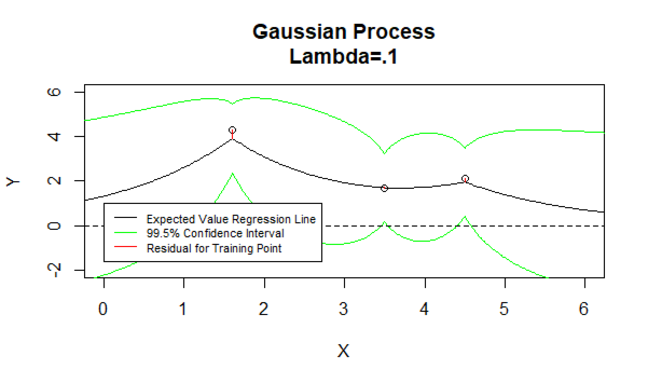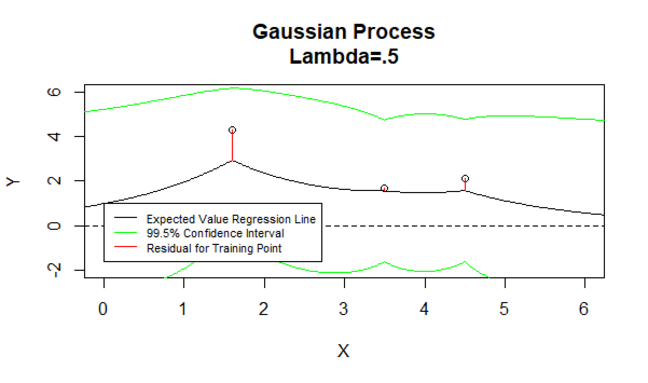We see that variance no longer drops to 0 at training points. Treating the expected value as a regression estimate, we also see that the model now has residuals (errors) on the training data. This is because the expected values at these points are dragged towards the process mean.

## Reversion to Mean

An interesting characteristic of Gaussian processes is that outside the training data they will revert to the process mean. The speed of this reversion is governed by the kernel used. This contrasts with many non-linear models which experience ‘wild’ behaviour outside the training data - shooting of to implausibly large values. An example is given with polynomial regression below (ignore the fact that the polynomial regression model is wildly overfitted, wild behaviour outside the training data can happen even on models that are not overfitted):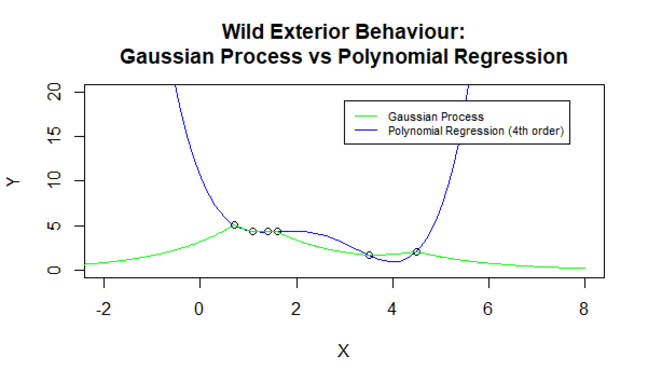## Training

When working with Gaussian processes, you will need to try a variety of kernels and values for the $$\lambda$$ hyper-parameter. Additionally, the kernels themselves will typically have a number of parameters that need to be specified and which function as hyper-parameters for the Guassian process training algorithm.

Typically you would decide on values for these hyper-parameters using a validation method. So as to make sure you select a good kernel function, you would be seeking to optimize the likelihood of the validation data rather than, for example, the mean squared error of the validation data to the expected value regression curve.

## Online Learning

Online learning is when the model updates itself to incoming data. It is simple to perform online learning with Gaussian processes. As new labelled data cases become available, the process covariance matrix is updated.

## Distribution over Functions

Consider that we can obtain conditional distributions for an arbitrary number of new $$X$$ values at once. Conceptually, we can even consider the case where we work with uncountably many new $$X$$ values, allowing us to obtain a conditional distribution for every point in the input space, given the training data. This conditional distribution is a distribution over a function on the input space.

Below are two examples where we sample a function from a Gaussian process generated from the training data:

$$X$$ $$Y$$
4.5 2.1
1.6 4.3
3.5 1.7
1.4 4.4
1.1 4.3
0.7 5.1

In the first case we generate one function, in the second we generate five. Note that the functions spend almost all their time within the 99.5% confidence intervals - as they should!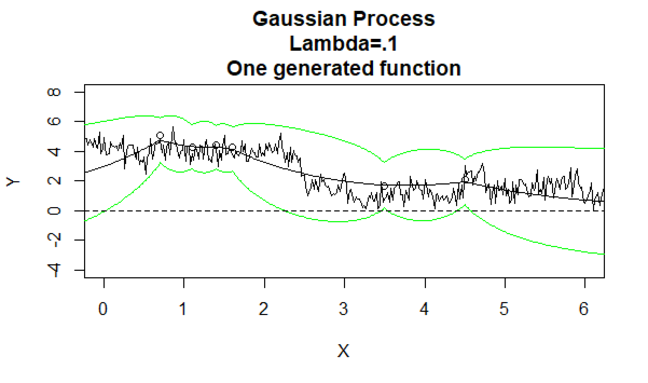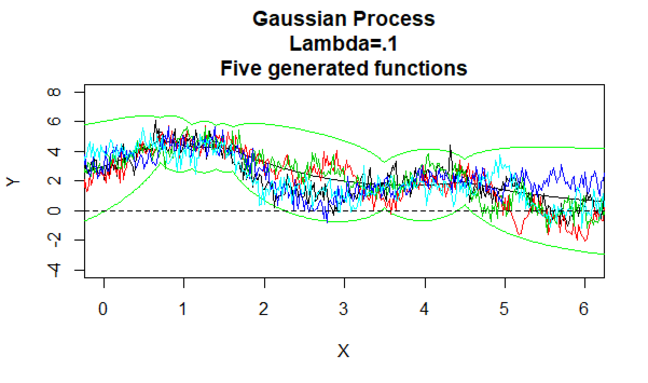Of course, we haven’t really sampled infinitely many points from an infinite covariance matrix. Rather we have generated a covariance matrix from the training data and a sequence of 300 $$X$$ values covering the plotted space, then sampled either once or five times from the resulting 300-variable multivariate conditional distribution. Then we join the 300 estimated $$Y$$ values for each sample.

Gaussian processes are used as distributions over functions in Bayesian non-parametric statistics.

## Scalability

Gaussian processes scale to large data even worse than most other kernel methods. We often have to calculate the kernel matrix in kernel methods, with a complexity of $$N^2$$ for a data set with $$N$$ rows. But for Gaussian processes, we have to also calculate the inverse of this matrix, leading to a complexity of $$N^3$$.

There are a number of ways of reducing the complexity by using approximations to either the covariance matrix or the inverse, such as approximating the inverse using numerical methods, or approximating the covariance matrix using a banded matrix and then approximating the inverse of this banded matrix by another banded matrix. We do not know how popular these approximation methods are in real life, but do think it fair to say that Gaussian processes are typically used with reasonably small data sets.